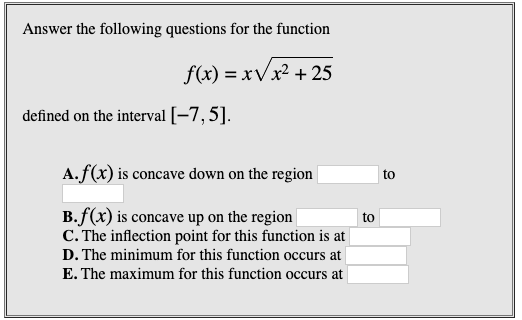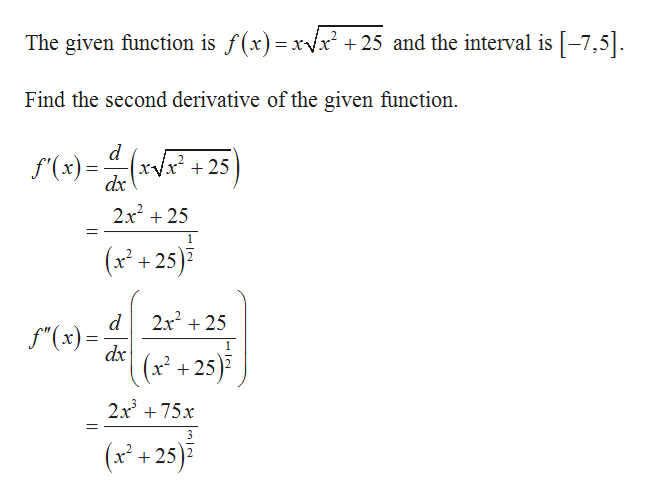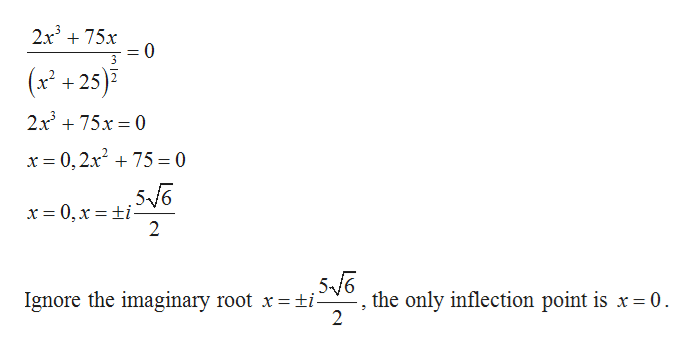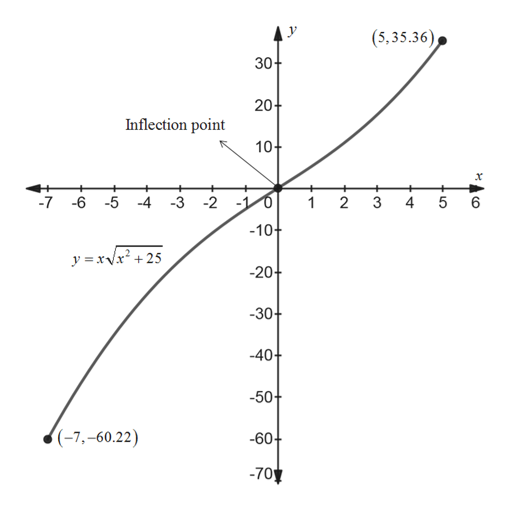# Answer the following questions for the functionf(x) = xVx² + 25defined on the interval [-7,5].A.f(x) is concave down on the regiontoB.f(x) is concave up on the regionC. The inflection point for this function is attoD. The minimum for this function occurs atE. The maximum for this function occurs at

Question
6 viewshelp_outlineImage TranscriptioncloseAnswer the following questions for the function f(x) = xVx² + 25 defined on the interval [-7,5]. A.f(x) is concave down on the region to B.f(x) is concave up on the region C. The inflection point for this function is at to D. The minimum for this function occurs at E. The maximum for this function occurs at fullscreen
check_circle

Step 1help_outlineImage TranscriptioncloseThe given function is f(x)=xvx² + 25 and the interval is [-7,5]. Find the second derivative of the given function. f(x) = (xVx* + 25 xyx + 25 dx %3D 2x + 25 (x² + 25)? 2x + 25 f"(x) = dx (x² + 25)? 2x + 75x (x² + 25)? fullscreen
Step 2

Equate the second derivative to zero to find the critical point.help_outlineImage Transcriptionclose2x + 75x %3D 0 = (x² +25)? 2x + 75x = 0 x = 0, 2x² + 75 = 0 x = 0, x = ±i- the only inflection point is x = 0. 2 Ignore the imaginary root x=±i- fullscreen
Step 3

Sketch the graph of the functio...help_outlineImage Transcriptionclose(5,35.36), 30- 20- Inflection point 10+ -4 -3 -2 -10 1 2 -7 -6 -5 4 -10+ y =xVx? + 25 -20+ -30- -40+ -50+ {(-7,-60.22) -60+ -70 15 fullscreen

### Want to see the full answer?

See Solution

#### Want to see this answer and more?

Solutions are written by subject experts who are available 24/7. Questions are typically answered within 1 hour.*

See Solution
*Response times may vary by subject and question.
Tagged in
MathCalculus

### Other